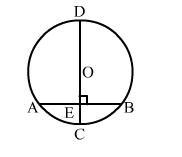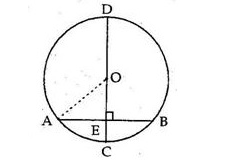# In the given figure, the diameter CD of a circle with centre O is perpendicular to chord AB.

Question:

In the given figure, the diameter CD of a circle with centre O is perpendicular to chord AB. If AB = 12 cm and CE = 3 cm, calculate the radius of the circle.Solution:

CD is the diameter of the circle with centre O and is perpendicular to chord AB.
Join OA.Given: AB = 12 cm and CE = 3 cm
Let OA = OC r cm   (Radii of a circle)
Then OE = (r - 3) cm
Since the perpendicular from the centre of the circle to a chord bisects the chord, we have:

$A E=\left(\frac{A B}{2}\right)=\left(\frac{12}{2}\right) \mathrm{cm}=6 \mathrm{~cm}$

Now, in right angled ΔOEA, we have:

$\Rightarrow O A^{2}=O E^{2}+A E^{2}$

$\Rightarrow r^{2}=(r-3)^{2}+6^{2}$

$\Rightarrow r^{2}=r^{2}-6 r+9+36$

$\Rightarrow r^{2}-r^{2}+6 r=45$

⇒ 6r = 45

$\Rightarrow r=\left(\frac{45}{6}\right) \mathrm{cm}=7.5 \mathrm{~cm}$

⇒ r = 7.5 cm
Hence, the required radius of the circle is 7.5 cm.